# How VFD Saves Power & Basic Concept of VFD

## How VFD Saves Power & Basic Concept of VFD:

VFD is nothing but a variable frequency drive, sometime it is called as VVFD (Variable Voltage Frequency drive). In an induction motor you can control speed of the motor by three methods. First one is Stator input voltage control, as the motor’s input voltage changes simultaneously motor speed also get changed. The second one is Pole changing method. You know the speed of the motor N is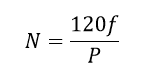From this equation, the speed of the motor N is indirectly proportional to the motor’s pole, therefore by changing the pole motor we can vary the speed of the motor. Third method is changing the frequency: From the motor speed equation, the speed of the motor is directly proportional to the input frequency of the motor this method is normally established by electronics types of equipment such as converter, inverter etc.

## The basic concept of VFD:

From our third method, we can change the speed of the motor by adjusting input frequency. This method gives smooth operation and accurate speed control of an induction motor.

But one important thing has to be taken care, while changing input supply frequency of the motor. Lete see the detailed explanation,

As we know the output emf of an alternator or generator E is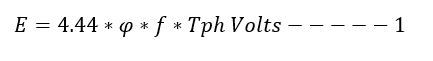In this,

E- emf in volts

Φ- is the flux in Weber

f- frequency in Hertz

Tph is the number of turns in armature (alternator/generator) or Transformer’s primary number turns.

In this, flux ϕ is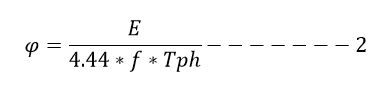By removing constants,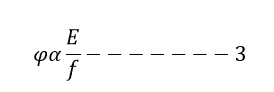From equation 3 the flux in the stator winding is directly proportional to the motor input voltage and indirectly proportional to the frequency of the motor. Hence while changing the frequency of the motor leads to changes in the flux, this may result into saturation of stator and the rotor cores. Such a saturation leads to sharp increase in the load current of the motor. So that we have to maintain the flux constant in the stator winding.

To achieve this, you can see the other variable E, the input voltage of the motor, along with changing the frequency, the voltage also must be changed. By doing that we can maintain constant E/f ratio. To ensures constant flux giving speed control without affecting the performance of the motor. Hence this method is called V/f control.

## Key Points: how VFD saves Power

As we know the input power P is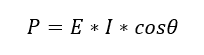The reducing the input voltage to the motor saves our energy, this is the concept behind the VFD

Variable voltage and variable frequency can be delivered by modern electronics scheme such as converter and inverter module.

Normally, the grid supply is available in constant voltage and constant frequency format typically AC voltage. In this, the converter consists of diode module or thyristor combinations are used to convert the input alternating current voltage to Direct current voltage. The input to the VFD is given through the line inductor, here inductor is used to protect our equipment from voltage spike. The output of the converter is given to inverter module through DC capacitors, which work as filter as well high voltage protector.

The inverter inverts DC voltage to AC variable voltage and variable frequency, by selecting the required frequency (speed) the output voltage of the inverter also varies and keeps E/f ratio constant. It ensures the smooth operation and speed control of an induction motor.

### Pole changing method:

We cannot maintain contactor logic; it is very complex circuit. Even I had worked for six months in pole changing speed changer, but it was difficult to work. If one phase of the contactor got stuck, then the motor takes high input current, due to this high current there is a changes of motor’s winding failure. In my carrier, two pole changing speed controller motors got burnt.

Also we cannot get exact speed of the motor. i.e the possible speed changes is 3000 rpm, 1500rpm, 750rpm, 325rpm, and so on. In between numbers we cannot get from this method.

Power saving is not possible as the input voltage is constant.

### Stator voltage control:

In this method, we can change the speed of the motor by adjusting the input voltage to the motor, The voltage variation can be done with the help of rheostat. In this method, due to reduction in the voltage, current drawn by the motor increases. Large changes in the voltage for small change in speed. This is the biggest disadvantage of this method. Due to decrease in voltage motor get overheated and the Power loss is very high. Hence this method is very rarely used in practice.

• Power saving
• Smooth operation
• Exact speed control
• Less maintenance
• Reliable operation

• Very costly
• The output voltage of the VFDs cannot be used to another load, so we have to provide separate VFDs for each motor
• Very costly especially IDBTs
• Needs technically skilled people to do annual maintenance
• Very sensitive electronic circuit boards, change of failure in our body static electricity

### IGBTs Pictures: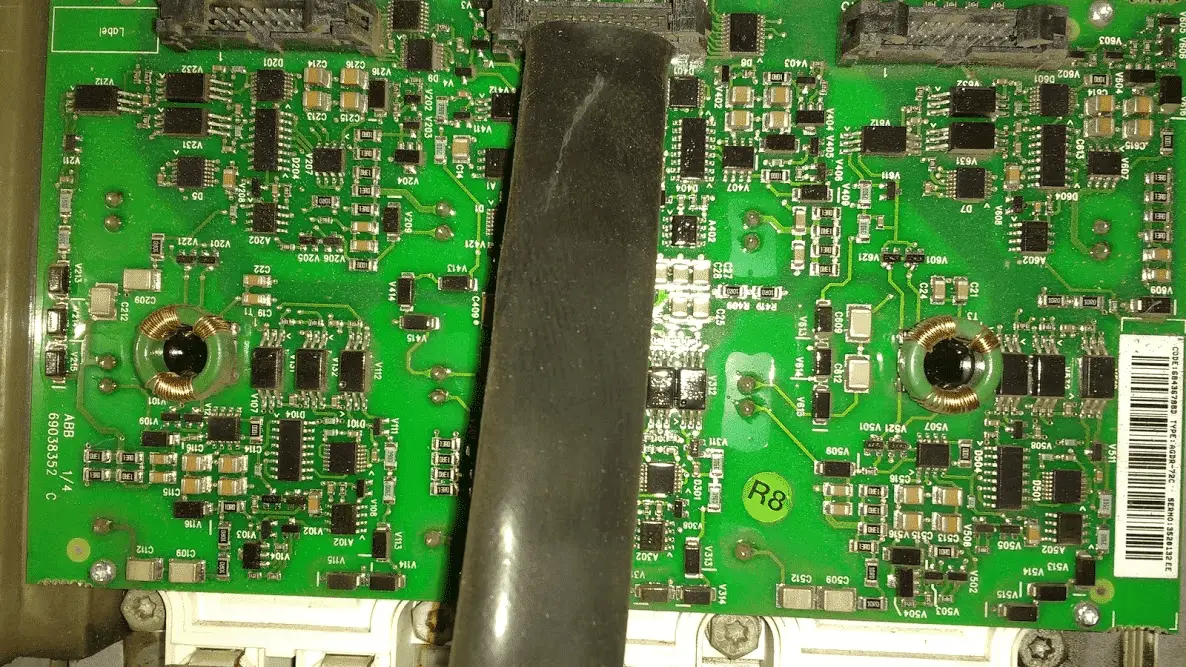#### 1 COMMENT

1. The picture is Abb make IGBT card if that gets fault how to identify it and not the AINT card